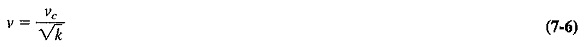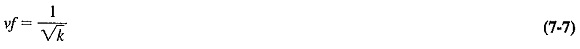## Losses in Transmission Lines:

Types of Losses in Transmission Lines are three ways in which energy, applied to a transmission line, may become dissipated before reaching the load: radiation, conductor heating and dielectric heating.

Radiation losses occur because a transmission line may act as an antenna if the separation of the conductors is an appreciable fraction of a wavelength. This applies more to parallel-wire lines than to coaxial lines. Radiation losses are difficult to estimate, being normally measured rather than calculated. They increase with frequency for any given transmission line, eventually ending that line’s usefulness at some high frequency.

Conductor heating, or I2R loss, is proportional to current and therefore inversely proportional to characteristic impedance. It also increases with frequency, this time because of the skin effect. Dielectric heating is proportional to the voltage across the dielectric and hence inversely proportional to the characteristic impedance for any power transmitted. It again increases with frequency (for solid dielectric lines) because of gradually worsening properties with increasing frequency for any given dielectric medium. For air, however, dielectric heating remains negligible.

Since the last two losses are proportional to length, they are usually lumped together and given by manufacturers in charts, expressed in decibels per 100 meters. For practical coaxial lines at 1 GHz, these losses vary from as much as 200 dB/100 m for a solid-dielectric, flexible 6-mm line, to as little as 0.94 dB/100 m for a rigid, air-dielectric 127-mm line.

### Velocity factor:

The velocity of light and all other electromagnetic waves depends on the medium through which they travel. It is very nearly 3 x 108 m/s in a vacuum and slower in all other media. The velocity of light in a medium is given bywhere

ν = velocity in the medium

νc = velocity of light in a vacuum

k = dielectric constant of the medium (1 for a vacuum and very nearly 1 for air)

The velocity factor of a dielectric substance, and thus of a cable, is the velocity reduction ratio and is therefore given byThe dielectric constants of materials commonly used in transmission lines range from about 1.2 to 2.8, giving corresponding velocity factors from 0.9 to 0.6. Note also that since ν = fλ and f is constant, the wavelength λ is also reduced by a ratio equal to the velocity factor. This is of particular importance in stub calculations. If a section of 300-Ω twin lead has a velocity factor of 0.82, the speed of energy transferred is 18 percent slower than in a vacuum.

Scroll to Top